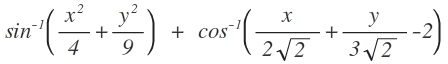Geometry Level 5If the value of the given expression is a, then what is $\lfloor a \rfloor$?

Details And Assumptions:

1. $x,y$ are cartesian plane co-ordinates.

2. Expression given here is a real-valued one, not complex-valued.

3. $sin^{-1}x,cos^{-1}x$ are defined on $[-1,1]$

×